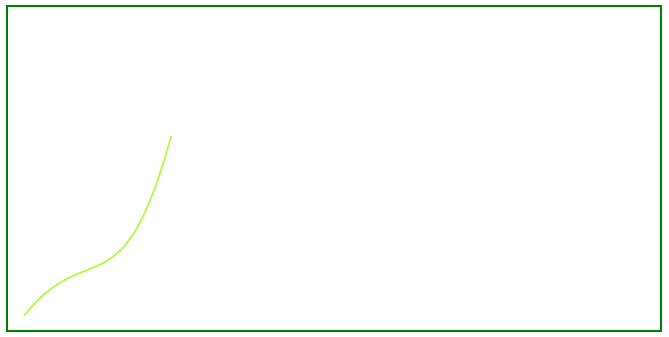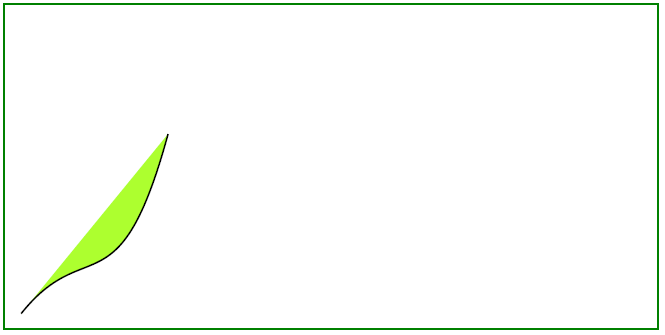# Add Curve Object to PDF file

Contents
[ ]

A graph Curve is a connected union of projective lines, each line meeting three others in ordinary double points.

Bézier curves are widely used in computer graphics to model smooth curves. The curve is completely contained in the convex hull of its control points, the points may be graphically displayed and used to manipulate the curve intuitively. The entire curve is contained in the quadrilateral whose corners are the four given points (their convex hull).

In this article, we will investigate simply graph curves, and filled curves, that you can create in your PDF document.

1. Create Document instance

2. Create Drawing object with certain dimensions

3. Set Border for Drawing object

4. Add Graph object to paragraphs collection of page

5. Save our PDF file

``````void ExampleCurve() {

// Create Document instance
// Create Document instance
auto document = MakeObject<Document>();

// Add page to pages collection of PDF file

// Create Drawing object with certain dimensions
auto graph = MakeObject<Aspose::Pdf::Drawing::Graph>(400, 200);

// Set border for Drawing object
auto borderInfo = MakeObject<BorderInfo>(BorderSide::All, Color::get_Green());
graph->set_Border(borderInfo);

auto curve1 = MakeObject<Aspose::Pdf::Drawing::Curve>(MakeArray<double> ({ 10, 10, 50, 60, 70, 10, 100, 120}));
curve1->get_GraphInfo()->set_Color(Color::get_GreenYellow());

// Add Graph object to paragraphs collection of page

// Save PDF file
}
``````

The following picture shows the result executed with our code snippet:## Create Filled Curve Object

This example shows how to add a Curve object that is filled with color.

``````void ExampleFilledCurve() {

// Create Document instance
// Create Document instance
auto document = MakeObject<Document>();

// Add page to pages collection of PDF file

// Create Drawing object with certain dimensions
auto graph = MakeObject<Aspose::Pdf::Drawing::Graph>(400, 200);

// Set border for Drawing object
auto borderInfo = MakeObject<BorderInfo>(BorderSide::All, Color::get_Green());
graph->set_Border(borderInfo);

auto curve1 = MakeObject<Aspose::Pdf::Drawing::Curve>(MakeArray<double>({ 10, 10, 50, 60, 70, 10, 100, 120}));
curve1->get_GraphInfo()->set_FillColor(Color::get_GreenYellow());